Function Repository Resource:

# NewtonCompanionMatrix

Generate the companion matrix for the Newton interpolating polynomial of a given set of points

Contributed by: Jan Mangaldan
 ResourceFunction["NewtonCompanionMatrix"][{f1,f2,…}] yields the Newton companion matrix of an interpolating polynomial which reproduces the function values fi at successive integer values 1, 2, …. ResourceFunction["NewtonCompanionMatrix"][{{x1,f1},{x2,f2},…}] yields the Newton companion matrix of an interpolating polynomial for the function values fi corresponding to the abscissas xi. ResourceFunction["NewtonCompanionMatrix"][{{{x1},f1,df1,…},…}] yields the Newton companion matrix of an interpolating polynomial that reproduces derivatives as well as function values.

## Details

ResourceFunction["NewtonCompanionMatrix"][data] gives an upper Hessenberg matrix whose characteristic polynomial is a scalar multiple of InterpolatingPolynomial[data,x].
The result of ResourceFunction["NewtonCompanionMatrix"] is a SparseArray.
With a list of data of length n, ResourceFunction["NewtonCompanionMatrix"] gives a square matrix with dimensions less than or equal to n-1.

## Examples

### Basic Examples (2)

Construct the Newton companion matrix for the squares:

 In:=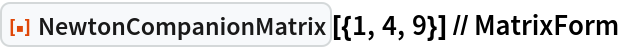Out=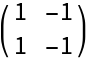Check its characteristic polynomial:

 In:=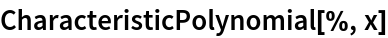Out=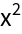Construct the Newton companion matrix corresponding to an interpolating polynomial through three points:

 In:=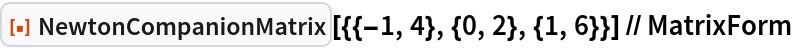Out=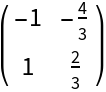The characteristic polynomial is a scalar multiple of the corresponding interpolating polynomial:

 In:=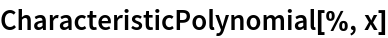Out=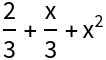In:=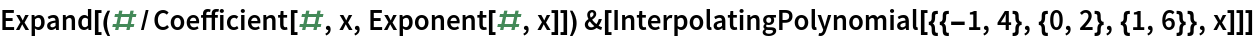Out=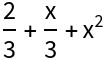### Scope (2)

Newton companion matrix for symbolic points:

 In:=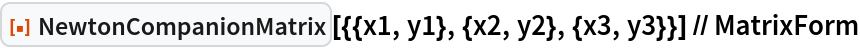Out=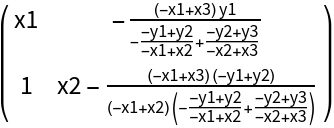Construct the Newton companion matrix for points with derivative information supplied:

 In:=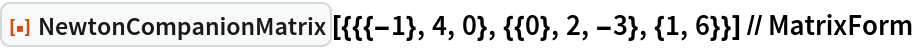Out=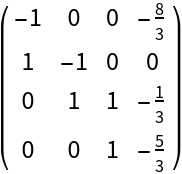### Applications (2)

Find the roots of an interpolating polynomial by computing the eigenvalues of a Newton companion matrix:

 In:=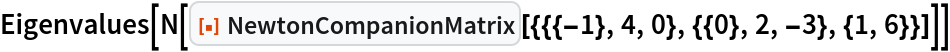Out=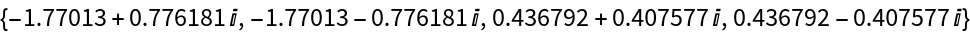Compare with the result of using NSolve:

 In:=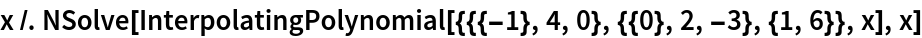Out=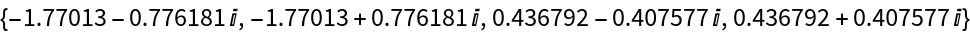### Properties and Relations (2)

The dimensions of a Newton companion matrix might be much less than the number of points supplied:

 In:=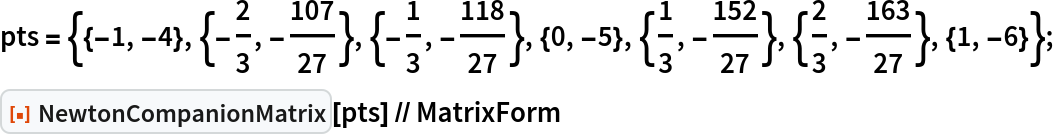Out=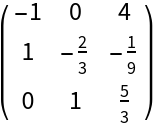In:=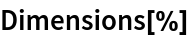Out=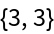In this case, the corresponding interpolating polynomial has degree 3:

 In:=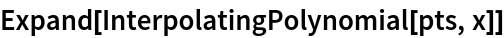Out=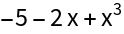## Version History

• 1.0.0 – 26 April 2021